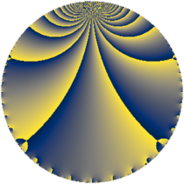# Properties

 Label 784.4.iLevel $784$ Weight $4$ Character orbit 784.i Rep. character $\chi_{784}(177,\cdot)$ Character field $\Q(\zeta_{3})$ Dimension $116$ Sturm bound $448$

# Related objects

## Defining parameters

 Level: $$N$$ $$=$$ $$784 = 2^{4} \cdot 7^{2}$$ Weight: $$k$$ $$=$$ $$4$$ Character orbit: $$[\chi]$$ $$=$$ 784.i (of order $$3$$ and degree $$2$$) Character conductor: $$\operatorname{cond}(\chi)$$ $$=$$ $$7$$ Character field: $$\Q(\zeta_{3})$$ Sturm bound: $$448$$

## Dimensions

The following table gives the dimensions of various subspaces of $$M_{4}(784, [\chi])$$.

Total New Old
Modular forms 720 124 596
Cusp forms 624 116 508
Eisenstein series 96 8 88

## Trace form

 $$116q + 5q^{3} + q^{5} - 485q^{9} + O(q^{10})$$ $$116q + 5q^{3} + q^{5} - 485q^{9} + 19q^{11} + 4q^{13} + 62q^{15} + q^{17} + 203q^{19} + 121q^{23} - 1165q^{25} - 382q^{27} - 352q^{29} - 307q^{31} - 65q^{33} + 9q^{37} + 734q^{39} - 292q^{41} - 1136q^{43} - 442q^{45} - 573q^{47} - 71q^{51} - 179q^{53} + 58q^{55} + 1802q^{57} + 1405q^{59} + 101q^{61} - 478q^{65} - 1105q^{67} - 3122q^{69} + 1920q^{71} + 1093q^{73} + 780q^{75} - 1183q^{79} - 3378q^{81} - 3848q^{83} + 2334q^{85} - 2350q^{87} + 645q^{89} - 859q^{93} + 1311q^{95} + 2332q^{97} + 8516q^{99} + O(q^{100})$$

## Decomposition of $$S_{4}^{\mathrm{new}}(784, [\chi])$$ into newform subspaces

The newforms in this space have not yet been added to the LMFDB.

## Decomposition of $$S_{4}^{\mathrm{old}}(784, [\chi])$$ into lower level spaces

$$S_{4}^{\mathrm{old}}(784, [\chi]) \cong$$ $$S_{4}^{\mathrm{new}}(7, [\chi])$$$$^{\oplus 10}$$$$\oplus$$$$S_{4}^{\mathrm{new}}(14, [\chi])$$$$^{\oplus 8}$$$$\oplus$$$$S_{4}^{\mathrm{new}}(28, [\chi])$$$$^{\oplus 6}$$$$\oplus$$$$S_{4}^{\mathrm{new}}(49, [\chi])$$$$^{\oplus 5}$$$$\oplus$$$$S_{4}^{\mathrm{new}}(56, [\chi])$$$$^{\oplus 4}$$$$\oplus$$$$S_{4}^{\mathrm{new}}(98, [\chi])$$$$^{\oplus 4}$$$$\oplus$$$$S_{4}^{\mathrm{new}}(112, [\chi])$$$$^{\oplus 2}$$$$\oplus$$$$S_{4}^{\mathrm{new}}(196, [\chi])$$$$^{\oplus 3}$$$$\oplus$$$$S_{4}^{\mathrm{new}}(392, [\chi])$$$$^{\oplus 2}$$